CBSE (Science) Class 11CBSE
Share

# Calculate the Oxidation Number of Sulphur, Chromium and Nitrogen in H2so5, Cr2o2−7cr2o72- and No−3no3-. Suggest Structure of These Compounds. Count for the Fallacy. - CBSE (Science) Class 11 - Chemistry

ConceptOxidation Number Balancing of Redox Reactions

#### Question

Calculate the oxidation number of sulphur, chromium and nitrogen in H2SO5, Cr_2O_7^(2-) and NO_3^-. Suggest structure of these compounds. Count for the fallacy.

#### Solution 1

O.N. of S in H2SO5. By conventional method, the O.N. of S in H2SO5 is 2 (+1) + x + 5 (-2) = 0 or x = +8 This is impossible because the maximum O.N. of S cannot be more than six since it has only six electrons in the valence shell. This fallacy is overcome if we calculate the O.N. of S by chemical bonding method. The structure of H2SOis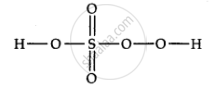2 x (+1) + x + 2(-1) + 3 x  (-2) = 0 or x = +6

(for H)       (for S)      for (O-O)     (for other O atoms

Cr in Cr_2O_7^(2-)

2(x) + (-2xx7) = -2

2x - 14 = -2

2x = -2 + 14 x = +6

x + 1(-1)  + 1(-2)     + 1(-2) = 0 or x + 5

(for O^-)   (for = O)  for ->O

Thus, there is no fallacy about the O.N. of N in N03whether one calculates by conventional method or by chemical bonding method.

#### Solution 2

i)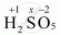2(+1) + 1(x) + 5(-2) = 0

=> 2 + x - 10 = 0

=>x = +8

However, the O.N. of S cannot be +8. S has six valence electrons. Therefore, the O.N. of S cannot be more than +6.

The structure of H2SO5 is shown as follows: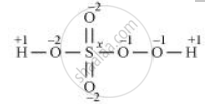Now, 2(+1) + 1(x) + 3(-2) + 2(-1) = 0

=> 2 + x - 6 - 2 = 0

=> x = +6

Therefore, the O.N. of S is +6.

ii)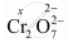2(x) + 7(-2) = -2

=> 2x - 14 = -2

=> x = +6

Here, there is no fallacy about the O.N. of Cr in Cr_2O_7^(2-).

The structure of  Cr_2O_7^(2-) is shown as follows: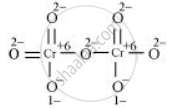Here, each of the two Cr atoms exhibits the O.N. of +6.

iii)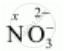1(x) + 3(-2) = -1

=> x - 6 = -1

=> x = +5

Here, there is no fallacy about the O.N. of N in NO_3^(-)

The structure of NO_3^(-) is shown as follows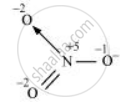The N atom exhibits the O.N. of +5.

Is there an error in this question or solution?

#### APPEARS IN

Solution Calculate the Oxidation Number of Sulphur, Chromium and Nitrogen in H2so5, Cr2o2−7cr2o72- and No−3no3-. Suggest Structure of These Compounds. Count for the Fallacy. Concept: Oxidation Number - Balancing of Redox Reactions.
S